## D.3 Programming Concepts within Arena

Within Arena , programming support comes in two forms: laying down flow chart modules and computer language integration (e.g. VBA, C, etc.). This section presents some common programming issues that are helpful to understand when trying to get the most out of your models. First, we will examine how Arena stores the output from a simulation run. Then, the discussion involving input and output started in Chapter 4 will be continued. Finally, the use of Visual Basic for Applications (VBA) within the environment will be introduced.

### D.3.1 Using the Generated Access File

Each time the simulation experiment is executed writes the statistical information collected during the run to a Microsoft Access database that has the same name as the model file. This database is what is used by the Crystal Reports report generator. You can also access this database and extract the statistical information yourself. This is useful if you need to post process the statistical information in a statistical package.

This section demonstrates how to extract information from this database. The intention is to make you aware of this database and to give you enough information so that you can use the database for some simple data extraction. There is detailed information concerning the Reports Database within the Help system. You should search under The Report Database. We will be reusing the LOTR example model from Chapter 4 within this section to illustrate these concepts.

When you create and run a model, e.g. yourmodel.doe, running the model will create a corresponding Microsoft Access file called yourmodel.mdb. Each time the model is run, the statistical information from the simulation is written to the database. If you change the input parameters to your model (without doing anything else), and then re-run the model, that model’s results are written to the database. Any previous data will be over written. There are two basic ways to save information from multiple simulation runs. The first is to simply rename the database file to a different name before rerunning the simulation. In this case, a new database file for the model will be created when it is run. This approach creates a new database for each simulation execution.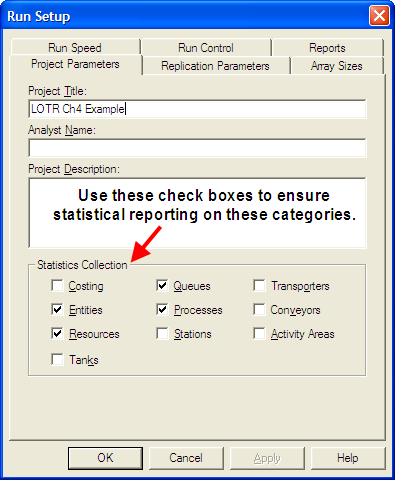Figure D.17: Run Setup statistical collection options

The second approach is to use the Projects Parameters panel within the Run $$>$$ Setup menu dialog as illustrated in Figure D.17. The Project Title field identifies a particular project. This field is used by the database to identify a given project’s results. If this name is not changed when the simulation is rerun then the results are overwritten for the named project. Thus, by changing this name before each experiment, the database will contain the information for each experiment accessible by this project name. Now let’s take a look at the type of information that is stored within the database.

The Arena Reports database consists of a number of tables and queries. A table is a set of records with each row in the table having a unique identifier called its primary key and a set of fields (columns) that hold information about the current record. A query is a database instruction for extracting particular information from a table or a set of related tables.

The help system describes in detail the structure of the database. For accessing statistical information there are a few key tables:

Definition

Holds the name of the statistical item from within the model, its type (e.g. DSTAT, TALLY, FREQ, etc.) and information about its collection and reporting.

Statistic

The summary within replication results for a given statistic on a given replication

Count

The ending value for a RECORD module designated as Count for a given replication.

Frequency

The ending values for tabulated frequency statistics by replication

Output

The value of a defined OUTPUT statistic for each replication.

The Definition table with the Statistic table can be used to access the replication information. Figure D.18 illustrates the fields within the Definition table. The important fields in the Definition table are ID, Name, and DefinitionTypeID. The ID is the primary key of the table, Name represents the name to appear on the report that was assigned within the model for the statistic, DefinitionTypeID indicates the type of statistical element. For the purposes of this section, the statistic types of interest are DSTAT (time-based), TALLY (observation-based), COUNTER (RECORD with count option), OUTPUT (captured at end of replication), and FREQUENCY (tabulates percentage of time spent in defined categories).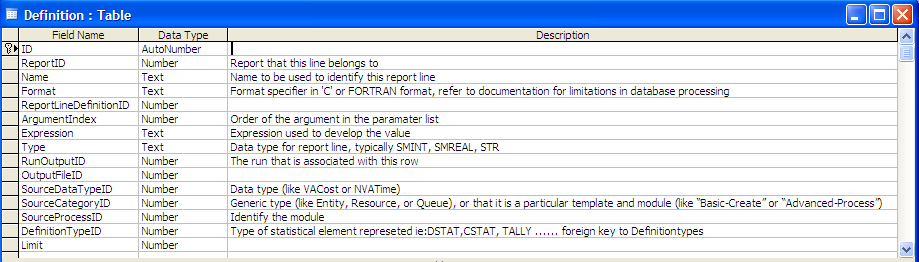Figure D.18: Definition table field design

The Statistic table holds the statistical values for the within replication statistics as shown in Figure D.19. Notice that the Statistic table has a field called DefintitionID. This field is a database foreign key to the Definition table. With this field you can relate the two tables together and get the name and type of statistic.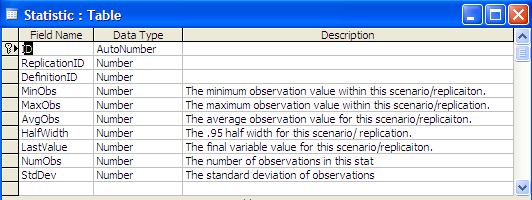Figure D.19: Statistic table field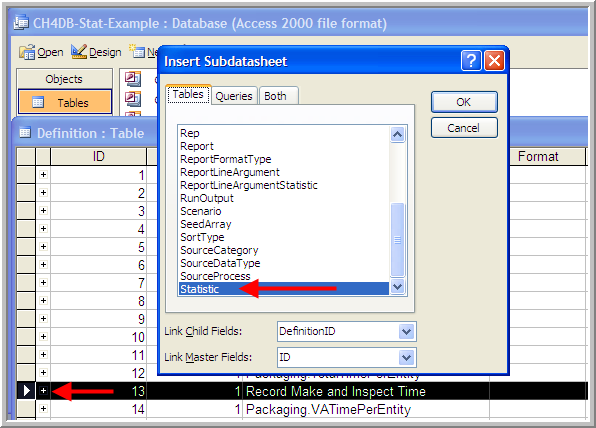Figure D.20: Accessing statistics information

To explore these tables, you will work with the database called DB-Stat-Example.mdb. This file was produced by running the LOTRExample.doe with 56 replications and renaming the created database file. If you have Microsoft Access then open the database and then open the table called Definition. Select the desired row, e.g. row 8, and use Insert $$>$$ Subdatasheet to get the Insert Subdatasheet dialog as shown in Figure D.20. Then, select the Statistic table and press the OK button as shown in the figure.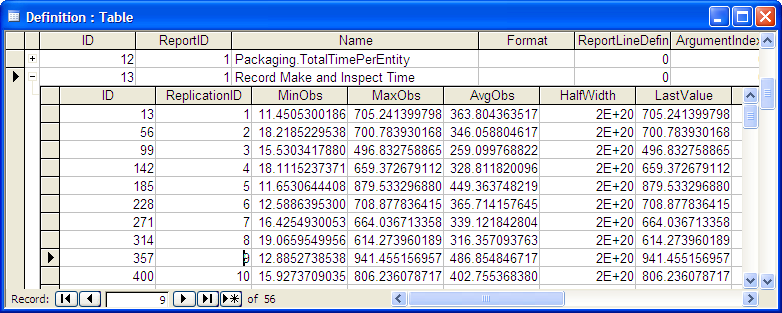Figure D.21: Replication statistics for make and inspect time

Figure D.21 shows the result of inserting the linked data sheet and selecting the “+" sign for the statistic named”Record Make and Inspect Time". You should right-click on the ReplicationID field and sort the subdatasheet by increasing replication number. From this table, you can readily assess the replication statistics. For example, the AvgObs column refers to the ending average across all the observations recorded during each replication. From this you can easily cut and paste any required information into a spreadsheet or a statistical package such as R. For those familiar with writing queries, this same information can be extracted by writing the query as shown in Figure D.22.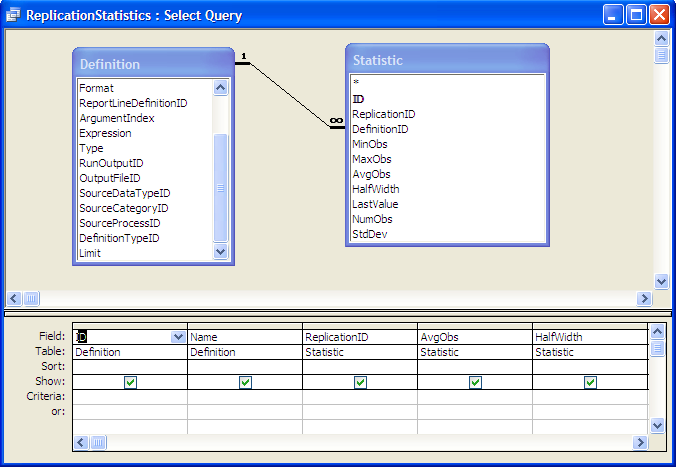Figure D.22: Query to access replication statistics

To summarize the statistics across the replications, you can write a group by query as shown in Figure D.23.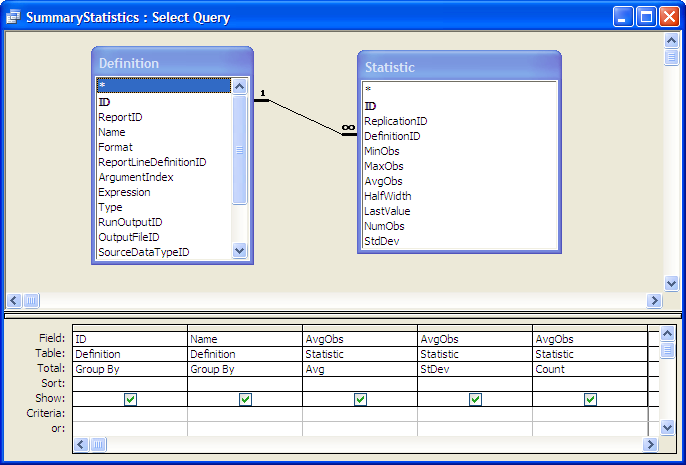Figure D.23: Query to summarize replication statistics

In the original LOTR Ring Maker, Inc. model, an OUTPUT statistic was defined to collect the time that the simulation ended. The OUTPUT statistic values collected at the end of each replication are saved in the Output table as shown in Figure D.24.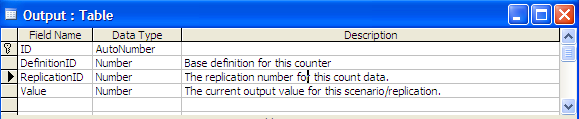Figure D.24: Output table field design

To see the collected values for a specific defined statistic, you can again use the Definition table. Open up the Definition table and scroll down to the row 48 which is the “TimeToMakeOrderStat” statistic. Select the row and using the Insert $$>$$ Subdatasheet dialog, insert the Output statistic table as the subsheet. You should see the subsheet as shown in Figure D.25. These same basic techniques can be used to examine information on the COUNTERS and FREQUENCY statistics that have been defined in the model. If you name each run with a different Project Name, then you can do queries across projects. Thus, after you have completed your simulation analysis you do not need to rerun your model, you can simply access the statistics that were collected from within the Reports database. If you are an experienced Microsoft Access user you can then form custom queries, reports, charts, etc. for your simulation results.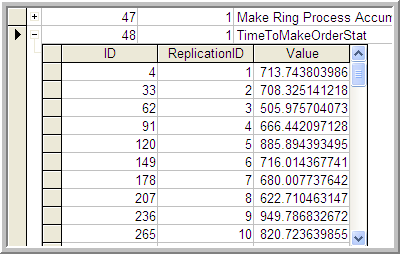Figure D.25: Expanded data sheet view for OUTPUT statistics

### D.3.2 Working with Files, Excel, and Access

#### D.3.2.1 Reading from a Text File

In this example, the SMART file, Smarts162.doe, is used to show how to read from a text file. Open up the file named Smarts162Revised.doe. Figure D.26 provides an overview of the model.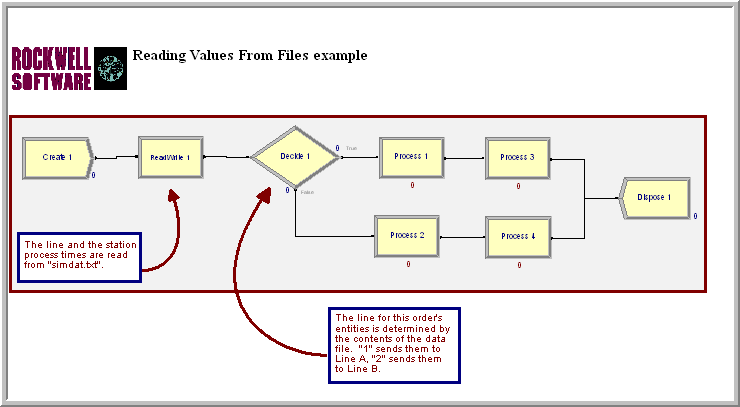Figure D.26: Smarts 162 Model

In this model, entities arrive according to a Poisson process, the type of entity and thus the resulting path through the processing is determined via the values within the simdat.txt file. The first number in the file is the type (1 or 2). Then, the following two numbers are the station 1 and station 2 processing times, as shown in Figure D.27. In Figure D.28, the READWRITE module reads directly from the SIMDAT file using a free format. Each time a read occurs, the attributes (myType, myStation1PT, myStation2PT) are read in. The myType attribute is tested in the DECIDE module and the attributes myStation1PT and myStation2PT are used in the PROCESS modules.Figure D.27: Sample processing times is simdat.txt

; This would be a comment followed by a header comment
;  Num Servers\ \ Arrival Rate
1 10

The Initialize option indicates what should do at the beginning of a replication. The file can stay at the current position (Hold), be rewound to the beginning of the file (Rewind), or the file can be closed (Close).

The rest of the model is straightforward. In this case, the values from the file are read into the attributes of an entity. The values of an array can also be read in using this technique.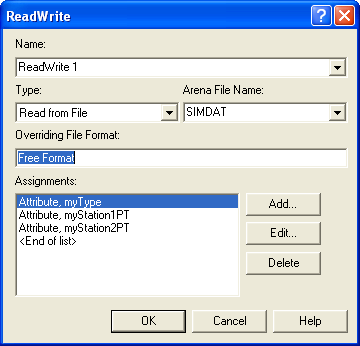Figure D.28: READWRITE module for simdat.txt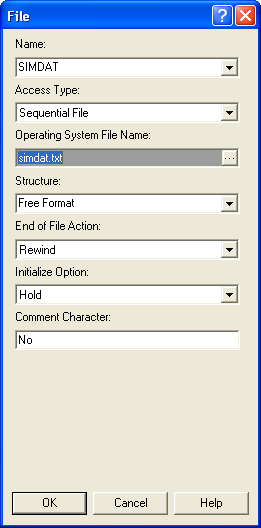Figure D.29: FILE module for Smarts162Revised.doe

#### D.3.2.2 Reading a Two Dimensional Array

Smarts file, Smarts164.doe, Figure D.30, shows how to read into a two-dimensional array. The CREATE module creates a single entity and the ASSIGN module initializes the array index. Then, iterative looping is performed using a DECIDE module as previously discussed in Chapter 4.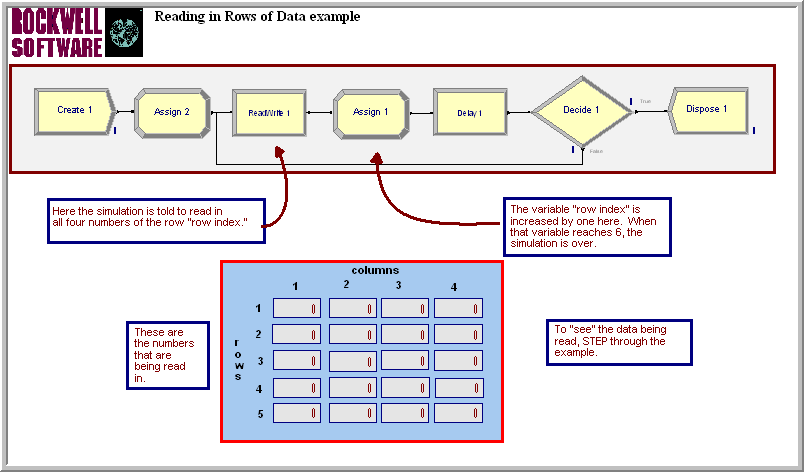Figure D.30: Smarts164.doe reading in a 2-D array

In this particular example, the entity delays for 10 minutes before looping to read in the next values for the array. The assignments for the READWRITE module are show in Figure D.31. You can easily see how the use of two WHILE-ENDWHILE loops could allow for reading in the size of the array. You would first read in the number of rows and columns and then loop through each row/column combination.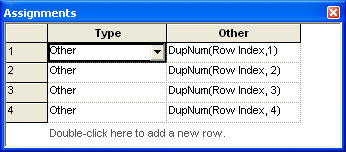Figure D.31: READWRITE assignments module using arrays

#### D.3.2.3 Reading from an Excel Named Range

So far the examples have focused on text files and for simple models these will often suffice. For more user friendly input of the data to files, you can read from Excel files. Smarts file 185, Figure D.32, shows how to read in values from an Excel named range.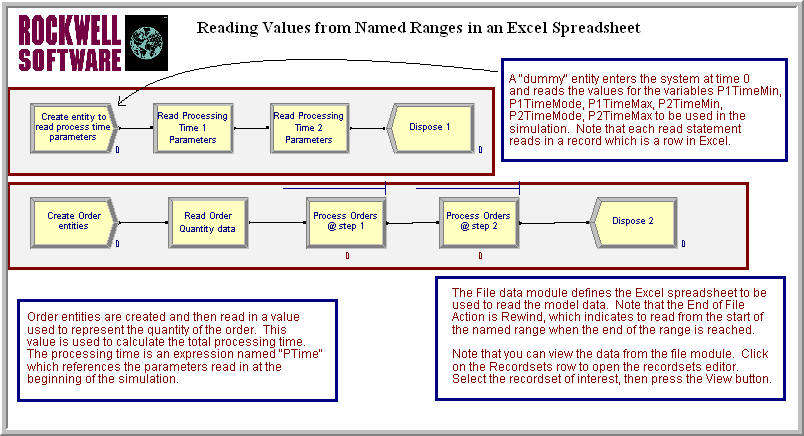Figure D.32: Smarts185.doe reading from an Excel named range

In order to read or write to an Excel named range, the named range must already be defined within the spreadsheet. Open up the Excel file Smarts185.xls. Select cells C5:E6 and look in the upper left hand corner of the Excel input bar. You will see the named range. By selecting any cells within Excel you can name them by typing the name into the named range box as indicated in Figure D.33. You can organize your spreadsheet in any way that facilitates the understanding of your data input requirements and then name the cells required for input. The named ranges are then accessible through the FILE module.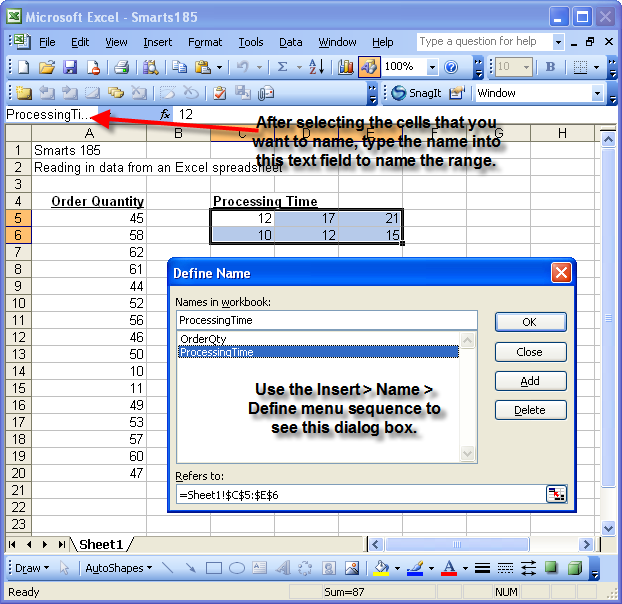Figure D.33: Checking the named range in Excel

In this example, the processing times are distributed according to a triangular distribution. The named spreadsheet cells hold the parameters of the triangular distribution (min, mode, max) for each of the two PROCESS modules. An EXPRESSION module was used to define expressions with the variables to be read in indicating the parameter values. The order quantities are how much the customer has ordered. The lower CREATE module creates 50 arriving customers, where the order quantity is read and then used in the processing times. In the use of named ranges, essentially the execution of each READWRITE module causes a new row to be read. Thus, as indicated in Figure D.32 there are two back to back READWRITE modules in the top level create logic to read in each of the rows associated with the processing times. In the bottom create logic, each new entity reads in a new row from the named range.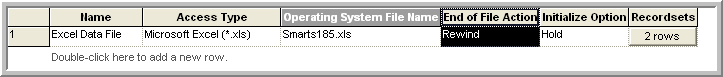Figure D.34: Data sheet view of FILE module

After setting up the spreadsheet and defining the named ranges within Excel, you must then a file with the named range. This is accomplished through the use of the FILE module. Select the FILE module within Smarts185.doe. The FILE module, Figure D.34, is already defined for you, but the steps will be indicated here. In the spreadsheet data view, double-click to add a new row and fill in AccessType, Operating System File Name, End of File Action, and Initialize Option the same as in the previous row. Then, click on the define Recordsets row button.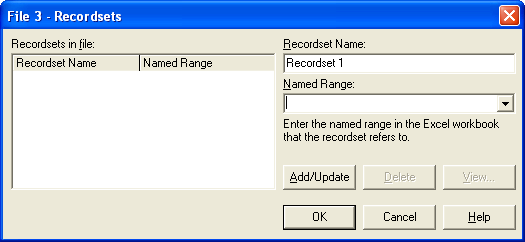Figure D.35: Defining the recordset for the FILE module

You will see a dialog box similar to Figure D.35. is smart enough to connect to Excel and to populate the Named Range text box with the named ranges that you defined for your spreadsheet. You need only select your desired named ranges and click on Add/Update. This will add the named range as a record set in the Recordsets in file area. You should add both named ranges as shown in Figure D.36. Once you select a named range you can also view the data in the range by selecting the View button. If you try this with the ProcessingTime named range, you will see a view of the data similar to that shown in Figure D.36. As you can see, it is very simple to define a named range and connect to it.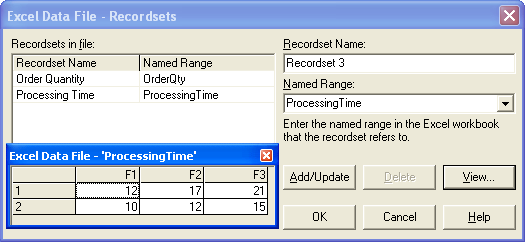Figure D.36: Viewing the ProcessingTime named range

Now, you have to indicate how to use the named range within the READWRITE module. Open up the READWRITE module, see Figure D.37, for reading the processing time 1 parameters. After selecting read from file and the associated file name, will automatically recognize the file as a named range based Excel file. You can then choose the recordset that you want to read from and then the module works essentially as it did in our text file examples. You can also use these procedures to write to Excel named ranges and to Access using active data objects (ADO). See for example SMARTS files 189 and 190.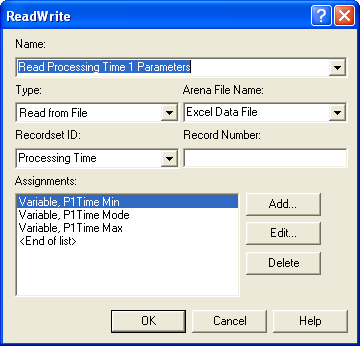Figure D.37: READWRITE module using named range

Making the simulation file driven takes special planning on how to organize the model to take advantage of the data input. Using sets and arrays appropriately is often necessary when making a model file driven. Here are some ideas for using files:

• Reading in the parameters of distributions

• Reading in model parameters (e.g. reorder points, order quantities, etc.)

• Reading in specified sequences for entities to follow. Define a set of sequences and read in the index into the set for the entity to follow. You can then define different sequences based on a file.

• Reading in different expressions to be used. Define a set of expressions and read in the index into the set to select the appropriate expression.

• Reading in a number of entities to create, then create them using a SEPARATE module.

• Creating entities and assigning their attributes based on a file

• Reading in each entity from a file to create a trace driven simulation

#### D.3.2.4 Reading Model Variables from Microsoft Access

This example uses the pharmacy model and augments it to allow the parameters of the simulation to be read in from a database. In addition, the simulation will write out statistical data at the end of each run. This example involves the use of Microsoft Access, if you do not have Access then just follow along with the text. In addition, the creation of the database show in Figure D.38 will not be discussed.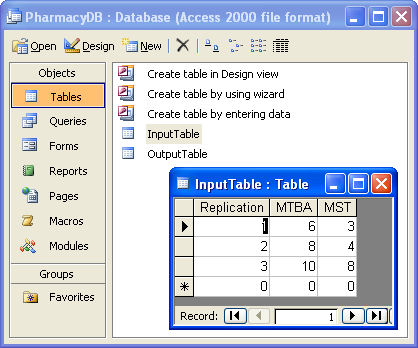Figure D.38: PharmacyDB Access Database

The database has two tables: one to hold the inputs and one to hold the output across simulation replications. Each row in the table InputTable has three fields. The first field indicates the current replication, the second field indicates the mean time between arrivals for that experiment and the last field indicates the mean service time for the experiment. This example will only have a total of 3 replications; however, each replication is not going to be the same. At the beginning of each replication, the parameters for the replication will be read in and then the replication will be executed. At the end of the replication, some of the statistics related to the simulation will be written out to the table called OuputTable. For simplicity, this table also only has three columns. The first column is the replication number, the second column will hold the average waiting time in the queue and the third column will hold the half-width reported from .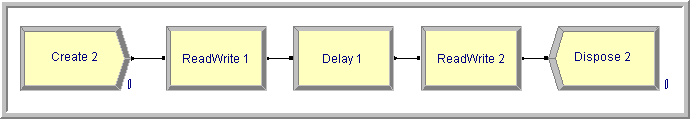Figure D.39: Read and write logic for Access example

Open up the PharmacyModelRWv1.doe file and examine the VARIABLE module. Notice that variables have been defined to hold the mean time between arrivals and the mean service time. Also, you should look at the CREATE and PROCESS modules to see how the variables are being used. The logic to read in the parameters at the beginning of each replication and to write the statistics at the end of the replication must now be implemented. The logic will be quite simple as indicated in Figure D.39. An entity should be created at time zero, read the parameters from the database, delay for the length of the simulation, and then write out the data.

You should lay down the modules indicated in Figure D.39. Now, the FILE module must be defined. The process for using Access as a data source is very similar to the way Excel named ranges operate. Figure D.40 shows the basic setup in the data sheet view. Opening up the recordset rows allows you to define the tables as recordsets. You should define the recordsets as indicated in the figure.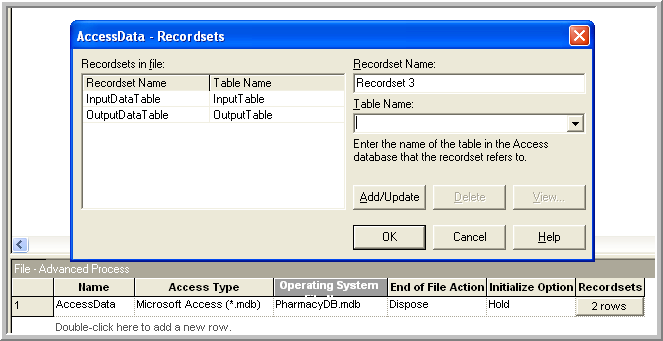Figure D.40: Read and write logic for Access example

The two READWRITE modules are quite simple. In the first READWRITE module (Figure D.41), the variables RepNum, MTBA, and MST are assigned values from the file. This will occur at time zero and thereby set the parameters for the run. The second READWRITE module (Figure D.42) writes out the value of the RepNum and uses the functions TAVG() and THALF() to get the values of the statistics associated with the waiting time in queue.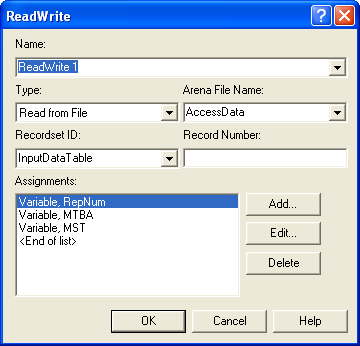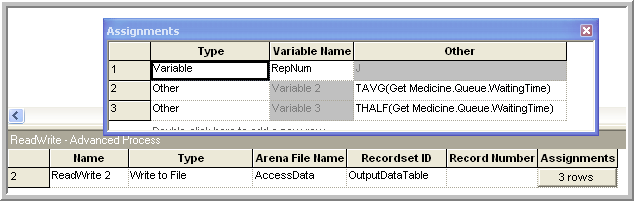Now, the setup of the replications must be considered. In this model, at the beginning of each replication the parameters will be read in. Since there are 3 rows in the database input table, the number of replications will be set to 3 so that all rows are read in. In addition, the run length is set to 1000 hours and the base time unit to minutes, as shown in Figure D.43.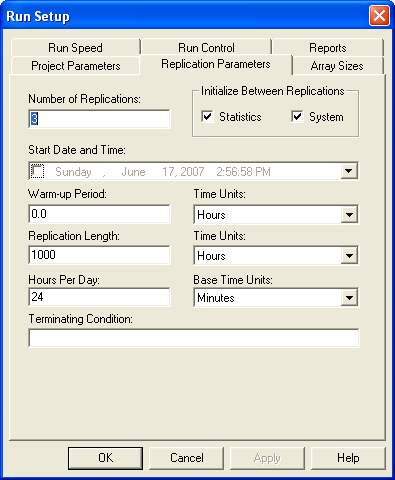Figure D.43: Run setup parameters for the database example

Only one final module is left to edit. Open up the DELAY module. See Figure D.44. The entity entering this module should delay for the length of the simulation. has a special purpose variable called TFIN which holds the length of the simulation in base time units. Thus, the entity should delay for TFIN units. Make sure that the units match the base time units specified in the Run Setup dialog.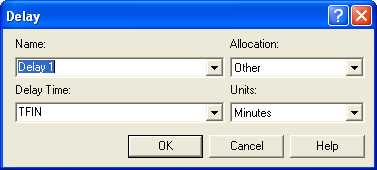Figure D.44: Delaying for the length of the replication

After the entity delays for TFIN time units, it enters the READWRITE module where it writes out the values of the statistics at that time. After running the model, you can open up the Microsoft Access database and view the OutputTable table. You should see the results for each of the three replications.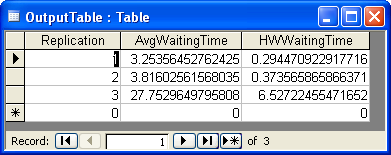Figure D.45: Output within the Access database

Suppose now you wanted to replicate each run involving the parameter settings 3 times. All you would need to do would be to set up your Access input table as shown in Figure D.45 and change the number of replications to 9 in the Run Setup dialog.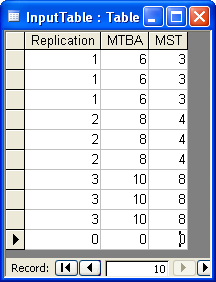Figure D.46: Making repeated replications

This same approach can be easily repeated for larger models. This allows you to specify a set of experiments, say according to an experimental design plan, execute the experiments and easily capture the output responses to a database. Then, you can use any of your favorite statistical analysis tools to analyze your experiments. I have found that for very large experiments where I want fine grained control over the inputs and outputs that the approach outlined here works quite nicely.

### D.3.3 Using Visual Basic for Applications

This section discusses the relationship between and Visual Basic for Applications (VBA). VBA is Microsoft’s macro language for applications built on top of the Windows operating system. As its name implies, VBA is based on the visual basic (VB) language. VBA is a full featured language that has all the aspects of modern computer languages include the ability to create objects with properties and methods. A full discussion of VBA is beyond the scope of this text. For an introduction to VBA for people interested in modeling, you might refer to .

The section assumes that the reader has some familiarity with VBA or is, at the very least, relatively computer language literate. This topic is relatively advanced and typical usage of often does not require the user to delve into VBA. The interaction between and VBA will be illustrated through a discussion of the VBA block and the use of Arena’s user defined function. Through VBA, also has the ability to create models (e.g. lay down modules, fill dialogs, etc) via Arena’s VBA automation model. This advanced topic is not discussed in this text, but extensive help is available on the topic via the on-line help system.

To understand the connection between and VBA, you must understand the user event oriented nature of VBA. Do not confuse the user interaction events discussed in this section with discrete events that occur within a simulation. Visual basic was developed as an augmentation of the BASIC computer language to facilitate the development of visual user interfaces. User interface interaction is inherently event driven. That is, the user causes various events to occur (e.g. move the mouse, clicks a button, etc.) that the user interface must handle. To build a program that interacts significantly with the user involves writing code to react to specific events. This paradigm is quite useful, and as VB became more accepted, the event-driven model of computing was expanded beyond that of directly interacting with the user. Additional non-user interaction events can be defined and can be called at specific places within the execution of the program. The programmer can then place code to be called at those specific times in order to affect the actions of the program. is integrated with VBA through a VBA event model.

There are a number of VBA events that are predefined within Arena’s VBA interaction model. The following key VBA events will be called automatically if an event routine is defined for these events in VBA within .

DocumentOpen, DocumentSave

These events are called when the model is opened or saved. The SIMAN object is not available, but the object can be used. The SIMAN object will be discussed within some examples.

RunBegin

This event is called prior to the model being checked. When the model is checked, the underlying SIMAN code is compiled and translated to executable machine code. You can place code within the RunBegin event to add or change modules with VBA automation so that the changes get complied into the model. The SIMAN object is not available, but the object can be used. This event occurs automatically when the user invokes a simulation run. The object will not be discussed in this text, but plenty of help is available within the help system.

RunBeginSimulation

This event is called prior to starting the simulation (i.e. before the first replication). This is a perfect location to set data that will be used by the model during all replications. The SIMAN object is active when this event is fired and remains active until after RunEndSimulation fires. Because the simulation is executing, changes using the object via automation are not permissible.

RunBeginReplication

This event is called prior to the start of each replication.

OnClearStatistics

This event is called if the clear statistics option has been selected in run setup. This event occurs prior to each replication and at the designated warm up time if a warm up period has been supplied.

RunEndReplication

This event is called at the end of each replication. It represents a perfect location for capturing replication statistical data. It is only called if the replication completes naturally with no interruption from errors or the user.

RunEndSimulation

This event is called after all replications are completed (i.e. after the last replication). It represents a perfect place to close files and get across replication statistical information.

RunPause, RunRestart, RunResume, RunStep, RunFastForward, RunBreak

These events occur when the user interacts with Arena’s run control (e.g. pauses the simulation via the pause button on the VCR control). These events are useful for prompting the user for input.

RunEnd

This event is called after RunEndSimulation and after the run has been ended. The SIMAN object is no longer active in this event.

These events are fired by the SIMAN runtime engine if the named event occurs.

UserFunction, UserRule

The UserFunction event is fired when the UF function is used within the model. The UserRule event is fired when the UR function is called within the model.

SimanError

This event is called if SIMAN encounters an error or warning. The modeler can use this event to trap SIMAN related errors.

A VBA event is defined, if a subroutine is written that corresponds to the VBA event naming convention within the ThisDocument module accessed through the VBA editor. In VBA, a file that holds code is called a module. This should not be confused with an module.

#### D.3.3.1 Using VBA

Let us take a look at how to write a VBA event for responding to the RunBegin event. The file, VBAEvents.doe that is available with this chapter, should be opened. The model is a simple CREATE, PROCESS, DISPOSE combination to create entities and have a model to illustrate VBA. The specifics of the model are not critical to the discussion here. In order to write a subroutine to handle the RunBegin event, you must use the VBA Editor. Within the environment use the Tools $$>$$ Macro $$>$$ Show Visual Basic Editor menu option (as shown in Figure D.47).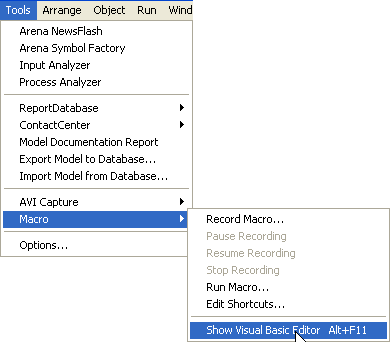Figure D.47: Showing the Visual Basic editor

This will open the VBA Editor as shown in Figure D.48. If you double click on the ThisDocument item in the VBA projects tree as illustrated in Figure D.48, you will open up a VBA module that is specifically associated with the current model.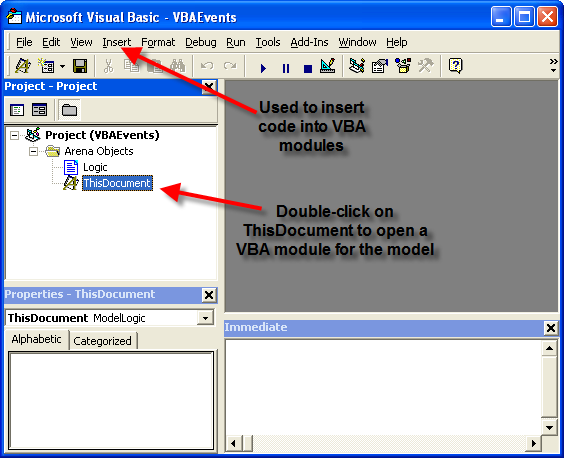Figure D.48: Showing the Visual Basic editor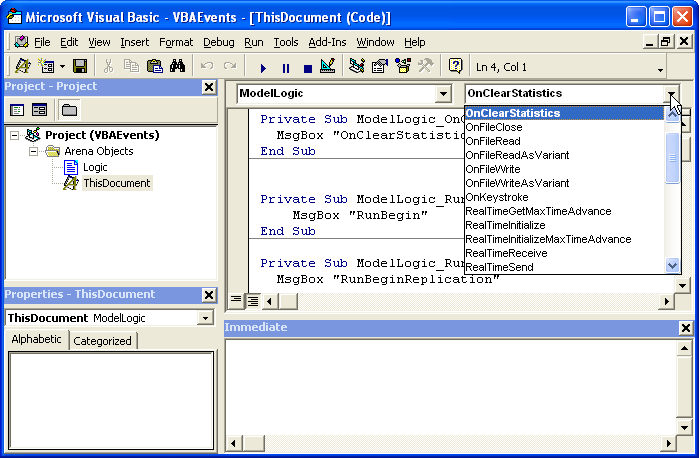Figure D.49: Showing the VBA Events for Arena

A number of VBA events (Figure D.49) have already been defined for the model. Let’s insert an event routine to handle the RunBegin event. Place your cursor on a line within the ThisDocument module and go to the event drop down box called (General). Within this drop down box, select Model Logic. Now go to the adjacent drop down box that lists the available VBA events. Clicking on this drop down list will reveal all the possible VBA events that are available, as shown in Figure D.49. The event routines that have already been written are in bold. As can be seen the OnClearStatistics event is indicating that it has been written and this can be confirmed by looking at the code associated with the ThisDocument module. At the end of the list is the RunBegin event. Select the RunBegin event and an event routine will automatically be placed within the ThisDocument module at your current cursor location.

The event procedure has a very special naming convention so that it can be properly called when the VBA integration mechanism needs to call it during the execution of the model. The code will be very simple, it will just open up a message box that indicates that the RunBegin VBA event has occurred. The code to open up a message box when the event procedure fires is as follows:

Private Sub ModelLogic_RunBegin()

MsgBox "RunBegin"

End Sub

The use of the MsgBox function in this example is just to illustrate that the subroutine is called at the proper time. You will quite naturally want to put more useful code within your VBA event subroutines.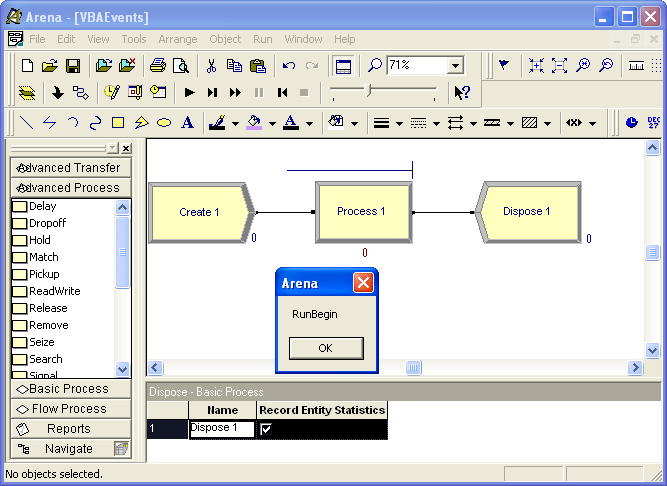Figure D.50: Message Box for RunBegin example

A number of similar VBA event subroutines have been defined with similar message boxes. Go back to the Environment and press the run button on the run control toolbar. As the model executes a series of message boxes will open up. After each message box appears, press okay and continue through the run. As you can see, the code in the VBA event routines is executed when fires the corresponding event. The use of the message box here is a bit annoying, but clearly indicates where in the sequence of the run that the event occurs.

The Smart files are a good source of examples related to VBA. The following models are located in the Smarts folder within your main folder (e.g., Program Files $$>$$ Rockwell Software $$>$$ Smarts).

• Smarts 001: VBA—VariableArray Value

• Smarts 016: Displaying a Userform

• Smarts 017: Interacting with Variables

• Smarts 024: Placing Modules and Filling in Data

• Smarts 028: Userform Interaction

• Smarts 081: Using a Shape Object

• Smarts 083: Ending a Model Run

• Smarts 086: Creating and Editing Resource Pictures

• Smarts 090: Manipulating a Module’s Repeat Groups

• Smarts 091: Creating Nested Submodels Via VBA

• Smarts 098: Manipulating Named Views

• Smarts 099: Populating a Module’s Repeat Group

• Smarts 100: Reading in Data from Excel

• Smarts 109: Accessing Information

• Smarts 121: Deleting a Module

• Smarts 132: Executing Module Data Transfer

• Smarts 142: VBA Submodels

• Smarts 143: VBA—Animation Status Variables

• Smarts 155: Changing and Editing Global Pictures

• Smarts 156: Grouping Objects

• Smarts 159: Changing an Entity Attribute

• Smarts 161: User Function

• Smarts 166: Inserting Entities into a Queue

• Smarts 167: Changing an Entity Picture

• Smarts 174: Reading/Writing Excel Using VBA Module

• Smarts 175: VBA Builds and Runs a Model

• Smarts 176: Manipulating Arrays

• Smarts 179: Playing Multimedia Files Within a Model

• Smarts 182: Changing Model Data Interactively

The Smarts files 001, 024, 090, 099, and 109 are especially illuminating. In the next section, the UserFunction event and the VBA block are illustrated.

#### D.3.3.2 The VBA Module and User Defined Functions

This section presents how to 1) use a user form to set the value of a variable, 2) call a user defined function which uses the value of an attribute to compute a quantity, and 3) use a VBA block to display information at a particular point in the model. Since the purpose of this example is to illustrate VBA, the model is a simple single server queuing system as illustrated in Figure D.51. The model consists of CREATE, ASSIGN, PROCESS, VBA, and DISPOSE modules.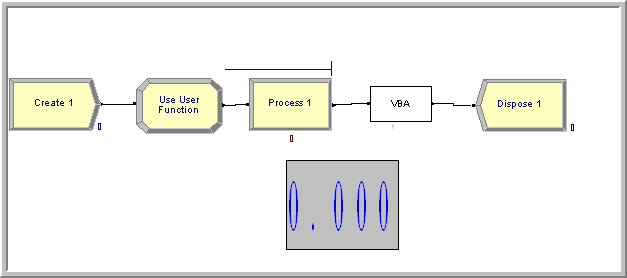Figure D.51: Simple VBA example model

The VBA block is found on the Blocks panel. To attach the Blocks panel, you can right-click in the Basic Process Panel area and select Attach, and then find the Blocks.tpo file. Now you are ready to lay down the modules as shown in Figure D.51. The information for each module is given as follows:

• CREATE: Choose Random(expo) with mean time between arrivals of 1 hour and set the maximum number of entities to 5.

• ASSIGN: Use and attribute called myPT and assign a U(10,20) random number to it via the UNIF(10,20) function.

• PROCESS: Use the SEIZE, DELAY, RELEASE option. Define a resource and seize 1 unit of the resource. In the Delay Type area, choose Expression and type in UF(1) for the expression.

• Using the VARIABLE data module to define a variable called vPTFactor and initialize it with the value 1.0.

No editing is necessary for the VBA block and the DISPOSE module. If you have any difficulty completing these steps you can look at the module dialogues in the file called VBAExample.doe.

Now you are ready to enter the world of VBA. Using Alt-F11, open the VBA Editor. Double-click on the ThisDocument object and enter the code as shown in the following code. If you don’t want to retype this code, then you can access it in the file VBAExample.doe.

Option Explicit

'' Declare global object variables to refer
'' to the Model and SIMAN objects
'' Public allows them to be accessed anywhere in this module
'' or any other vba module
Public gModelObj As Model
Public gSIMANObj As SIMAN

'' Variables can be accessed via their uniquely assigned
'' symbol number. An integer variable is needed to hold the index
'' It is declared public here so it can be used throughout this module
'' and other vba modules
Public vPTFactorIndex As Integer

'' Index for the myPT attribute
'' It is declared private here so it can be used throughout this module
Private myPTIndex As Integer

Let’s walk through what this code means. The two public variables gModelObj and gSIMANObj are object reference variables of type Model and SIMAN respectively. These variables allow access to the properties and methods of these two objects once the object references have been set. The Model object is part of Arena’s VBA Object model and allows access to the features of the Model as a whole. The details of Arena’s Object model can be found within the help system by searching on Automation Programmer’s Reference. The SIMAN object is created after the simulation has been complied and essentially gives access to the underlying simulation engine. The variables vPTFactorIndex and myPTIndex will be used to index into SIMAN to access the variable vPTFactor and the attribute myPT.

This example will use VBA forms to get some input from the user and to also display some information. Thus, the forms to be used in the example need to be developed. Use Insert $$>$$ UserForm to create two forms called Interact and UserForm1 as shown in Figure D.52.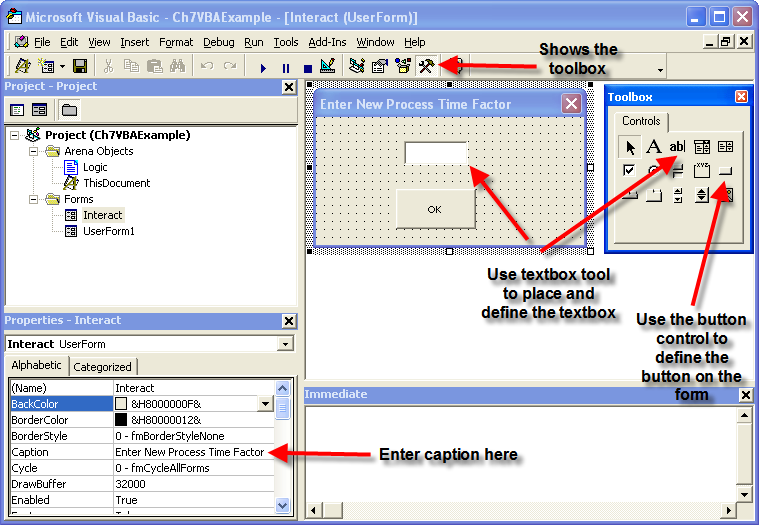Figure D.52: Building the Interact form

Use the show toolbox button to show the VBA controls toolbox. Then, you can select the desired control and place your cursor on the form at the desired location to complete the action. Build the forms as shown in the Figure D.52 and Figure D.53. To place the labels used in the forms, use the label control (right next to the textbox control on the Toolbox). The name of a form can be changed in the Misc $$>$$ (Name) property as shown in Figure D.54. Now that the forms have been built, the controls on the forms can be referenced within other VBA modules.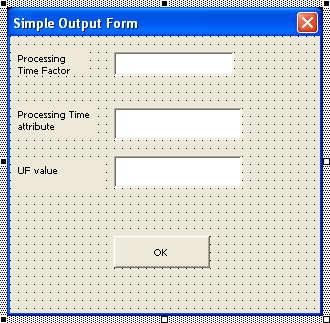Figure D.53: VBA UserForm1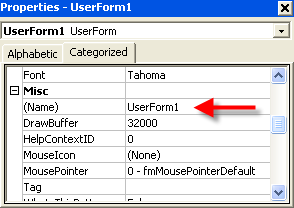Figure D.54: Properties Window

Before looking at the code for this situation, we need to understand how to interchange data between the model and the VBA code. This will be accomplished using the SIMAN object within the VBA environment. When a model is complied each element of the model is numbered. This is called the symbol number. The method, SymbolNumber(element name), on the SIMAN object will return the index symbol number that can be used as an identifier for the named element. To find information on the SIMAN object search the help system on SIMAN Object (Automation). There are many properties and methods associated with the SIMAN Object. The documentation states the following concerning the SymbolNumber method.

SymbolNumber Method

Syntax SymbolNumber (symbolString As String, index1 As Long, index2 As Long) As Long

Description All defined simulation elements have a unique number. For those constructs that have names, this function may be used to return the number corresponding to the construct name. For example, many of the methods in the SIMAN Object require an argument like resourceNumber or variableNumber, etc. to identify the specific element. Since it is more common to know the name rather than the number of the item, SymbolNumber(“MyElementName”) is often used for the elementNumber type argument.

As indicated in the documentation, an important part of utilizing the SIMAN object’s other methods is to have access to an element’s unique symbol number. The symbol number is an integer and is then used as an index into other method calls to identify the element of interest. According to the help system the VariableArrayValue property can be used either to get or to set the value associated with the variable identified by the index number.

Syntax VariableArrayValue (variableNumber As Long) As Double

Description Gets/Sets the value of the variable, where variableNumber is the instance number of the variable as listed in the VARIABLES Element. For more information on working with variables in automation, please see making variable assignments.

Now we are ready to look at the code. The VBA code shows the RunBeginSimulation event. When the user executes the simulation, the RunBeginSimulation event is fired. The first two lines of the routine set the object references to the Model object and to the SIMAN object in order to store the values in the global variables that were previously defined for the VB module. The SIMAN object can then be accessed through the variable gSIMANObj to get the indexes of the attribute and variable myPT and vPTFactor. In the exhibit, after the indexes to the myPT and vPTFactor are found, the SIMAN object is used to access the VariableArrayValue property. In this code, the value of the variable is accessed and then assigned to the value of the TextBox1 control on the Interact form. Then, the Interact form is told to show itself and the focus is set to the text box, TextBox1, on the form for user entry.

Private Sub ModelLogic_RunBeginSimulation()
'' set object references
Set gModelObj = ThisDocument.Model
Set gSIMANObj = ThisDocument.Model.SIMAN

'' Get the index to the symbol number
'' if the symbol does not exist there will be an error,
'' There is no error handling in this example
'' The SymbolNumber method of the SIMAN object returns
'' the index associated with the named symbol

'' get the index for the myPT attribute
myPTIndex = gSIMANObj.SymbolNumber("myPT")

'' get the index for the vPTFactor variable
vPTFactorIndex = gSIMANObj.SymbolNumber("vPTFactor")

'' set the current value of the textbox to the
'' current value of the vPT variable in Arena
'' The VariableArrayValue method of the SIMAN object
'' returns the value of the variable associated with the index
Interact.TextBox1.value =
gSIMANObj.VariableArrayValue(vPTFactorIndex)

'' Display the user form
Interact.Show
'' Set the focus to the textbox
Interact.TextBox1.SetFocus

'' The code for setting the variable's value is
'' in the OK button user form code
End Sub

When the Interact form is displayed, the text box will show the current value of the variable. The user can then enter a new value in the text box and press the OK button. Since the user interacted with the OK button, the button press event within the VBA form code can be used to change the value of the variable within to what was entered by the user.

To enter the code to react to the button press, go to the Interact form and select the OK button on the form. Right-click on the button and from the context menu, select View Code. This will open up the form’s module and create a VBA event to react to the button click.

The code provided next shows the use of the VariableArrayValue property to assign the value entered into the textbox to the vPTFactor variable. To access a public variable within the ThisDocument module, you precede the variable with ThisDocument. (e.g. ThisDocument.gSIMANObj). Once the value of the text box has been assigned to the variable, the form is hidden and input focus is given back to the model via the ThisDocument.gModelObj.Activate method.

Private Sub CommandButton2_Click()
'' when the user clicks the ok button
'' Set the current value of vPT to the value
'' currently in the textbox
'' uses the global variable vPTFactorIndex
'' defined in module ThisDocument
'' uses the global variable for the SIMAN object
'' defined in ThisDocument

ThisDocument.gSIMANObj.VariableArrayValue(ThisDocument.vPTFactorIndex) = TextBox1.value

'' hide the form
Interact.hide
'' Tell the model to resume running
ThisDocument.gModelObj.Activate
End Sub

Since the setting of the variable vPTFactor occurs as a result of the code initiated by the RunBeginSimulation event the value supplied by the user will be used for the entire run (unless changed again within the model or within VBA).

The model will now begin to create entities and execute as a normal model. Each entity that is created will go through the ASSIGN module and have its myPT attribute set to a randomly drawn U(10,20) number. Then the entity proceeds to the PROCESS module where it invokes the SEIZE, DELAY, and RELEASE logic. In this module, the processing time is specified by a user defined function via the UF(fid) function. The UF(fid) function will call associated VBA code from directly within an model.

You should think of the UF(fid) function as a mechanism by which you can easily call VBA functions. The fid argument is an integer that will be passed into VBA. This argument can be used to select from other user written functions as necessary. The following code exhibit shows the code for the UF function for this example.

'' This Function allows you to pass a user
'' defined value back to the
'' module which called upon the UF(functionID) function in Arena.
'' Use the functionID to select the function that you want via
'' The functions can obviously be named something
'' more useful than UserFunctionX()
'' The functions must return a double
Private Function ModelLogic_UserFunction(ByVal entityID As Long,
ByVal functionID As Long) As Double
'' entityID is the active entity
'' functionID is supplied when the user calls UF(functionID)

Select Case functionID
Case 1
ModelLogic_UserFunction = UserFunction1()
Case 2
ModelLogic_UserFunction = UserFunction2()
End Select

End Function

When you use the UF function, you must write your own code. To write the UF function, use the drop down box that lists the available VBA events on the ThisDocument module and select the UserFunction event. The UserFunction event routine that is created has two arguments. The first argument is an identifier that represents the current active entity and the second argument is what was supplied by the call to UF(fid).

When you create the function it will not have any code. You should organize your code in a similar fashion. As can be seen in the exhibit, the supplied function identifier is used within a VBA select-case statement to select from other available user written functions.

By using this select-case construct, you can easily define a variety of your own functions and call whichever function you need from within the model by supplying the appropriate function identifier.

In order to implement the called functions, we need to understand how to access attributes associated with the active entity. This can be accomplished using two methods associated with the SIMAN object: ActiveEntity and AttributeValue. The help system describes the use of these methods as follows.

ActiveEntity Method

Syntax ActiveEntity () As Long

Description Returns the record location (the entity pointer) of the currently active entity, or 0 if there is not one. This is particularly useful in a VBA block Fire event to access attributes of the entity that entered the VBA block in the model.

AttributeValue Method

Syntax AttributeValue (entityLocation As Long, attributeNumber As Long, index1 As Long, index2 As Long) As Double

Description Returns the value of general-purpose attribute attributeNumber* with associated indices index1 and index2. The number of indices specified must match the number defined for the attribute.*

Let’s see how to put these methods into action within the user defined functions. The following code exhibit shows how to access the value of an attribute associated with the active entity.

Private Function UserFunction1() As Double
'' each entity has a unique id
Dim activeEntityID As Long
'' get the number of the active entity
'' this could have been passed from ModelLogic_UserFunction
activeEntityID = gSIMANObj.ActiveEntity

Dim PTvalue As Double
'' get the value of the myPT attribute
PTvalue = gSIMANObj.AttributeValue(activeEntityID, myPTIndex, 0, 0)

Dim factor As Double
factor = gSIMANObj.VariableArrayValue(vPTFactorIndex)

'' this could be complicated function of the attribute/variables
'' here it is very simple (and could have been done within Arena itself
'' rather than VBA
UserFunction1 = PTvalue * factor
End Function

First, the ActiveEntity property of the SIMAN object is used to get an identifier for the current active entity (i.e. the entity that entered the VBA block). Then, the AttributeValue method of the SIMAN object is used to get the value of the attribute.

Within Arena, attributes can be defined as multi-dimensional via the ATTRIBUTES module. Multi-dimensional attributes are not discussed in this text, but the AttributeValue method allows for this possibility. The two indices that can be supplied indicate to the SIMAN objecti how to access the attribute. In the example, these two values are zero, which indicates that this is not a multi-dimensional attribute. Once the values of the myPT attribute and the vPTFactor variable are retrieved from the SIMAN object, they are used to compute the value that is returned by the UF function. The variables factor and PTvalue are used to calculate the product of their values. Admittedly, this calculation can be more readily accomplished directly in without VBA, but the point is you can implement any complicated calculation using this architecture.

With the UF function, you can easily invoke VBA code from essentially any place within your model. The UF function is especially useful for returning a value back to the model. Using the VBA block, you can also invoke specific VBA code when the entity passes through the block. In this example, after the entity exits the PROCESS module, the entity enters a VBA block.

When you use a VBA block within your model, each VBA block is given a unique number. Then, within the ThisDocument module, an individual event routine can be created that is associated with each VBA block. In the example, the VBA block is used to open up a form and display some information about the entity when it reaches the VBA block. This might be useful to do when running an model in order to stop the model execution each time the entity goes through the VBA block to allow the user to interact with the form. However, most of the time the VBA block is used to execute complicated code that depends on the state of the system when the entity passes through the VBA block. The following exhibit shows the code for the VBA block event.

Private Sub VBA_Block_1_Fire()
'' set the values of the textboxes
UserForm1.TextBox1.value = gSIMANObj.VariableArrayValue(vPTFactorIndex)
UserForm1.TextBox2.value = gSIMANObj.AttributeValue(gSIMANObj.ActiveEntity,
myPTIndex, 0, 0)
UserForm1.TextBox3.value = UserFunction1()
'' Display the user form
UserForm1.Show
End Sub

By now, this code should start to look familiar. The SIMAN object is used to access the values of the attribute and the variable that are of interest and then set them equal to the values for the textboxes that are being used on the form. Then, the form is shown. This brings up the form so that the user can see the values. In UserForm1, a command button was defined to close the form. The logic for hiding the form is shown in the next exhibit.

Private Sub CommandButton1_Click()
'' hide the form
UserForm1.hide
'' Tell the model to resume running
ThisDocument.gModelObj.Activate
End Sub

The show and hide functionality of VBA forms are used within this example so that new instances of the forms did not have to be created each time they are used. This keeps the form in memory so that the controls on the form can be readily accessed. This is not necessarily the best practice for managing the forms, but allows the discussion to be simplified. As long as you don’t have a large number of forms, this approach is reasonable. In addition, within the examples, there is no error catching logic. VBA has a very useful error catching mechanism and professional code should check for and catch errors.

#### D.3.3.3 Generating Correlated Random Variates

The final example involves how to explicitly model dependence (correlation) within input distributions. In the fitting of input models, it was assumed and tested that the sample observations did not have correlation. But, what do you do if the data does have correlation? For example, let $$X_i$$ be the service time of the $$i^{th}$$ customer. What if the $$X_i$$ have significant correlation? That is, the service times are correlated. Arrival processes might also correlated. That is, the time between arrivals might be correlated. Research has shown, see and , that ignoring the correlation when it is in fact present can lead to gross underestimation of the actual performance estimates for the system.

The discussion here is based on the Normal-to-Anything Transformation as discussed in , , , and . Suppose you have a $$N(0,1)$$ random variable, $$Z_i$$, and a way to compute the CDF, $$\Phi(z)$$, of the normal distribution. Then, based on the inverse transform technique, the random variable, $$\Phi(Z_i)$$ will have a $$U(0,1)$$ distribution. Suppose that you wanted to generate a random variable $$X_i$$ with CDF $$F(x)$$, then you can use $$\Phi(Z_i)$$ as the source of uniform random numbers in an inverse transform technique.

$X_i = F^{-1}(U_i) = F^{-1}((\Phi(Z_i)))$

This transform is called the normal-to-anything (NORTA) transformation. It can be shown that even if the $$Z_i$$ are correlated (and thus so are the $$\Phi(Z_i)$$) then the $$X_i$$ will have the correct CDF and will also be correlated. Unfortunately, the correlation is not directly preserved in the transformation so that if the $$Z_i$$ have correlation $$\rho_z$$ then the $$X_i$$ will have $$\rho_x$$ (not necessarily the same). Thus, in order to induce correlation in the $$X_i$$ you must have a method to induce correlation in the $$Z_i$$.

One method to induce correlation in the $$Z_i$$ is to generate the $$Z_i$$ from an autoregressive time-series model of order 1, i.e. AR(1). An AR(1) model with $$N(0,1)$$ marginal distributions has the following form:

$Z_i = \phi Z_{i-1} + \varepsilon_i$

where $$Z_1 \sim N(0,1)$$, with independent and identically normally distributed errors, $$\varepsilon_i \sim N(0,1-\phi^2)$$ with $$-1<\phi<1$$ for . $$i = 2,3,\ldots$$.

It is relatively straightforward to generate this process by first generating $$Z_1$$, then generating $$\varepsilon_i \sim N(0,1-\phi^2)$$ and using $$Z_i$$ = $$\phi Z_{i-1} + \varepsilon_i$$ to compute the next $$Z_i$$. It can be shown that this AR(1) process will have lag-1 correlation:

$\phi = \rho^1 = \text{corr}(Z_i, Z_{i+1})$

Therefore, you can generate a random variable $$Z_i$$ that has a desired correlation and through the NORTA transformation produce $$X_i$$ that are correlated with the correlation being functionally related to $$\rho_z$$. By changing $$\phi$$, one can get the correlation for the $$X_i$$ that is desired. Procedures for accomplishing this are given in the previously mentioned references. The spreadsheet NORTAExample.xls can also be used to perform this search process.

The implementation of this technique cannot be readily achieved in a general way within through the use of standard modules. In order to implement this method, you can make use of VBA. The model, NORTA-VBA.doe, shows how to implement the NORTA algorithm with a user defined function. Just as illustrated in the last section, a user defined function can be written as shown in the following code exhibit.

Private Function ModelLogic_UserFunction(ByVal entityID As Long,
ByVal functionID As Long) As Double

Select Case functionID
Case 1
ModelLogic_UserFunction = CorrelatedUniform()
Case 2
Dim u As Double
u = CorrelatedUniform()
Dim x As Double
x = expoInvCDF(u, 2)
ModelLogic_UserFunction = x
End Select

End Function 

When you uses UF(1), a correlated U(0,1) random variable will be returned. If the user uses UF(2), then a correlated exponential distribution with a mean of 2 will be returned. Notice that in generating the correlated exponential random variable, a correlated uniform is first generated and then used in the inverse transform method to transform the uniform to the proper distribution. Thus, provided that you have functions that implement the inverse transform for the desired distributions, you can generate correlated random variables.

The following code exhibit illustrates how the NORTA technique is used to generate the correlated uniform random variables. The variable, phi, is used to control the resulting correlation in the AR(1) process. The function, SampleNormal, associated with the SIMAN object is used to generate a $$N(0,1)$$ random variable that represents the error in the AR(1) process.

Private Function CorrelatedUniform() As Double
Dim phi As Double
Dim e As Double
Dim u As Double
'' change phi inside this function to get a different correlation
phi = 0.8
'' generate error
e = gSimanObj.SampleNormal(0, 1 - (phi * phi), 1)
'' compute next AR(1) Z
mZ = phi * mZ + e
'' use normal cdf to get uniform
u = NORMDIST(mZ)
CorrelatedUniform = u
End Function

Private Function expoInvCDF(u As Double, mean As Double) As Double
expoInvCDF = -mean * Log(1 - u)
End Function

The variable, mZ, has been defined at the VBA module level, and thus it retains its value between invocations of the CorrelatedUniform function. The variable, mZ, represents the AR(1) process. The current value of mZ is multiplied with phi and the error is added to obtain the next value of mZ. The initial value of mZ was determined by implementing the RunBeginReplication event within the ThisDocument module. Because of the NORTA transformation, mZ, is $$N(0,1)$$, and the function NORMDIST is used to compute the probability associated with the supplied z-value. The NORMDIST function (not shown here) is an implementation of the CDF for the standard normal distribution.

With minor changes, the code supplied in NORTA-VBA.doe can be easily adapted to generate other correlated random variables. In addition, the use of the UF function can be expanded to generate other distributions that does not have built in (e.g. binomial).